Temporary Work _______ 20080324 from 200703 I have been working on the Prime number series since March 2007. After working on this project with so much work invested I did not want to end the project. The problem was that I had a parabola that described all values of the components of my equation. Even though the log spirals I formed were not true logarithmic spirals, the equation of the parabola was still useful. The only problem was that when solving for “x” I assumed that “y” equaled the Prime number. However there were some operations done to the equation before “x” was solved. This is where this work starts. I have found the new values for y that relate to “x” when the number is Prime. Hard to describe but it is easily seen in the algebraic equations that follow. This is important, but it is only a start. It has to be tested make sure it is not true for all values and unique to Primes. However, we now know where “x” is in relation to the parabola. Much work needs done.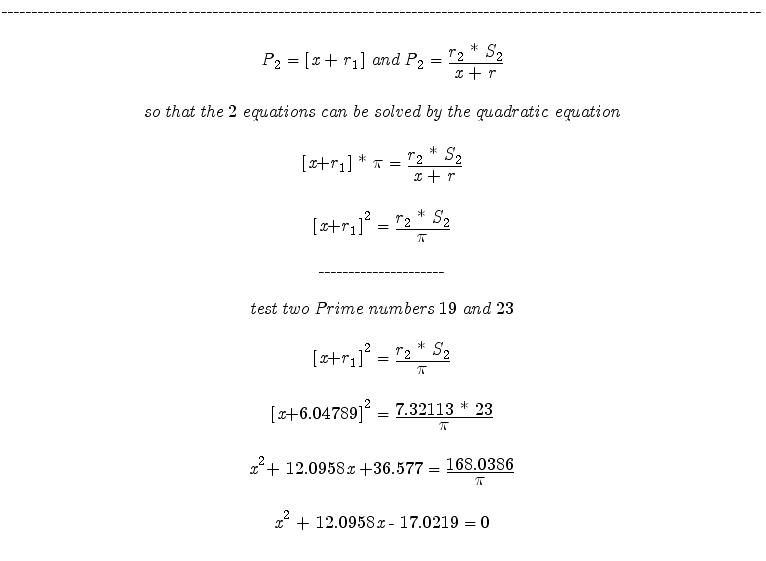We have changed the Prime number Y and must account for this: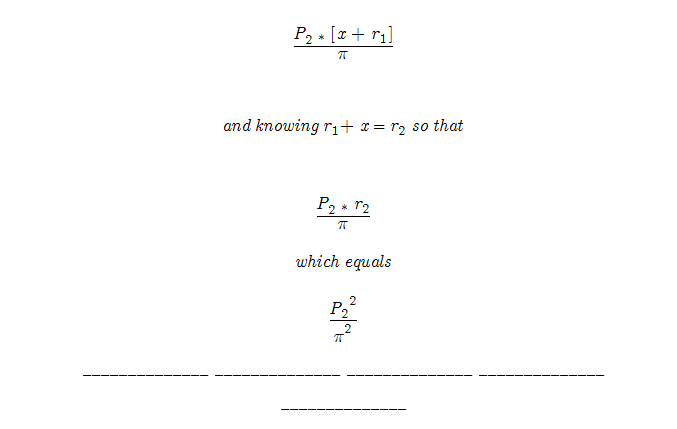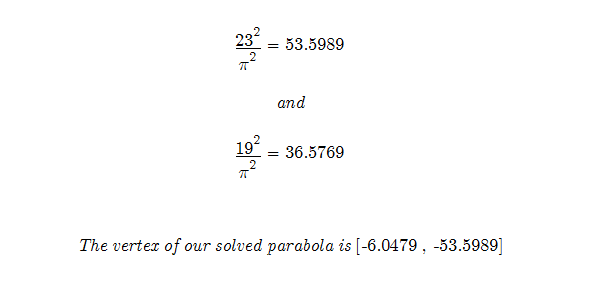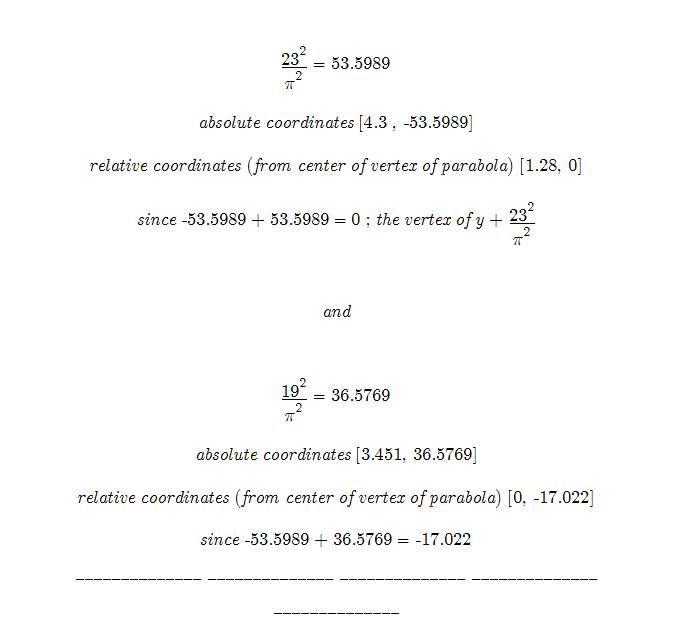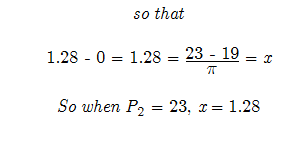May the Creative Force be with You ------- Hopefully it is clear what is being attempted to be solved here. I will post updates to better explain and hopefully solve this problem. This is a good group project. If you have read this and want to work on a problem email: trurlthe_constructor@hotmail.com . Also more math can be found in the math_hunches section of Constructor’s Corner.